Courses

# Test: Logarithm- 1

## 10 Questions MCQ Test Quantitative Aptitude (Quant) | Test: Logarithm- 1

Description
This mock test of Test: Logarithm- 1 for Quant helps you for every Quant entrance exam. This contains 10 Multiple Choice Questions for Quant Test: Logarithm- 1 (mcq) to study with solutions a complete question bank. The solved questions answers in this Test: Logarithm- 1 quiz give you a good mix of easy questions and tough questions. Quant students definitely take this Test: Logarithm- 1 exercise for a better result in the exam. You can find other Test: Logarithm- 1 extra questions, long questions & short questions for Quant on EduRev as well by searching above.
QUESTION: 1

### Which of the following statements is not correct?

Solution:

(a) Since loga a = 1, so log10 10 = 1.
(b) log (2 + 3) = log 5 and log (2 x 3) = log 6 = log 2 + log 3
∴ log (2 + 3) ≠ log (2 x 3)
(c) Since loga 1 = 0. so logio 1 = 0.
(d) log (1 + 2 + 3) = log 6 = log (1 x 2 x 3) = log 1 + log 2 + log 3.
So. (b) is incorrect.

QUESTION: 2

### If log 2 = 0.3010 and log 3 = 0.4771, the value of log5 512 is:

Solution: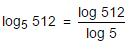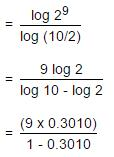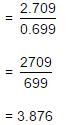QUESTION: 3

###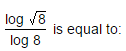Solution: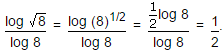QUESTION: 4

If log 27 = 1.431, then the value of log 9 is:

Solution: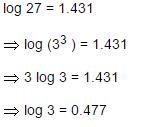∴ log 9 = log(32) = 2 log 3 = (2 x 0.477) = 0.954

QUESTION: 5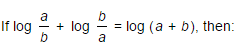Solution: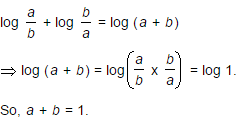QUESTION: 6

If log10 7 = a, then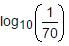is equal to :

Solution: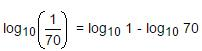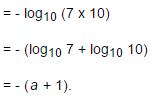QUESTION: 7

If log10 2 = 0.3010, then log2 10 is equal to:

Solution: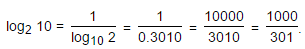QUESTION: 8

If log10 2 = 0.3010, the value of log10 80 is:

Solution: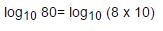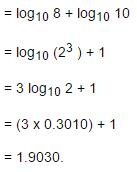QUESTION: 9

If log10 5 + log10 (5x + 1) = log10 (x + 5) + 1, then x is equal to:

Solution:

log10 5 + log10 (5x + 1) = log10 (x + 5) + 1

⇒ log10 5 + log10 (5x + 1) = log10 (x + 5) + log10 10

⇒ log10 [5 (5x + 1)] = log10 [10(x + 5)]

⇒ 5(5x + 1) = 10(x + 5)

⇒ 5x + 1 = 2x + 10

⇒ 3x = 9

⇒ x = 3.

QUESTION: 10

The value of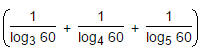is:

Solution:

Given expression= log60 3 + log60 4 + log60 5
= log60 (3 x 4 x 5)
= log60 60
= 1.